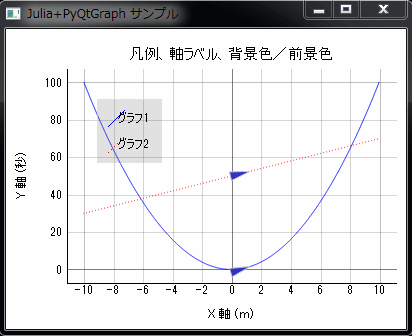# [PYTHON] Draw a graph with Julia + PyQtGraph (2)

"Draw a graph in the programming language Julia" I succeeded in moving the sample for the time being, but the data created by my own program (in Julia language) I decided to find out how to draw a graph using. I made a sample to display the legend / axis label and change the background color / foreground color (for printing). As usual, the calculation result obtained by Julia is displayed as a graph in PyQtGraph. (Please create an environment and move it)

• ArrowSample2.jl (Julia source code) passes an array to a function called graph_run () in the Python source. Let Python draw the graph for you. Calculate \$ y \$ of \$ y = x ^ 2 \$ and \$ y = 2x + 50 \$ in the range of \$ -10 ≤ x ≤ 10 \$ (\$ x \$ is in 0.1 increments </ del> 2000 pieces Divide into divisions).
• ArrowSample2.py (Python source code) draws a line graph based on the array passed by Julia.
• When calling Python code using PyCall, C: \ julia-0.2.1-win32 \ bin is included in the load path, so both ArrowSample2.jl and ArrowSample2.py are C: \ julia- Save it under 0.2.1-win32 \ bin.
• When saving the source code, the character encoding should be utf-8.
• To launch the sample, double-click ArrowSample2.jl (Julia source code) from Explorer.

Note: For the environment construction, refer to "Drawing a graph in the programming language Julia".#### `jl:ArrowSample2.jl(C:\julia-0.2.1-win32\Save to bin)`

``````
# -*- coding: utf-8 -*-

using PyCall

@pyimport ArrowSample2
x  = linspace(-10, 10, 2001)
y1 = [ x[i]*x[i] for i=1:length(x) ]
y2 = [ x[i]*2+50 for i=1:length(x) ]
ArrowSample2.graph_run(x, y1, y2)
``````

#### `python:ArrowSample2.py(C:\julia-0.2.1-win32\Save to bin)`

``````
# -*- coding: utf-8 -*-

from pyqtgraph.Qt import QtCore, QtGui
import pyqtgraph as pg

def graph_run(plot_data_x, plot_data_y1, plot_data_y2):

app = QtGui.QApplication([])

#Specifying antialiasing makes the plot cleaner
pg.setConfigOptions(antialias=True)
pg.setConfigOption('background', (255, 255, 255)) #Background = white
pg.setConfigOption('foreground', (0, 0, 0))       #Foreground = black

win = pg.GraphicsWindow(title=u'Julia+PyQtGraph sample')
win.resize(400,300)

mypen=pg.mkPen(color=(255, 0, 0), style=QtCore.Qt.DotLine) #Dotted line, red

p1 = win.addPlot(title=u'Legend, axis label, background color / foreground color')
p1.setLabel('left', u'Y axis', units=u'Seconds')
p1.setLabel('bottom', u'X axis', units=u'ｍ')
c1 = p1.plot(x=plot_data_x, y=plot_data_y1, pen=(0,0,255), name=u'Graph 1') #Solid line, blue
c2 = p1.plot(x=plot_data_x, y=plot_data_y2, pen=mypen, name=u'Graph 2')     #Dotted line, red
p1.showGrid(x=True, y=True) #Show grid

#Arrow animation(Graph 1)
a1 = pg.CurveArrow(c1)